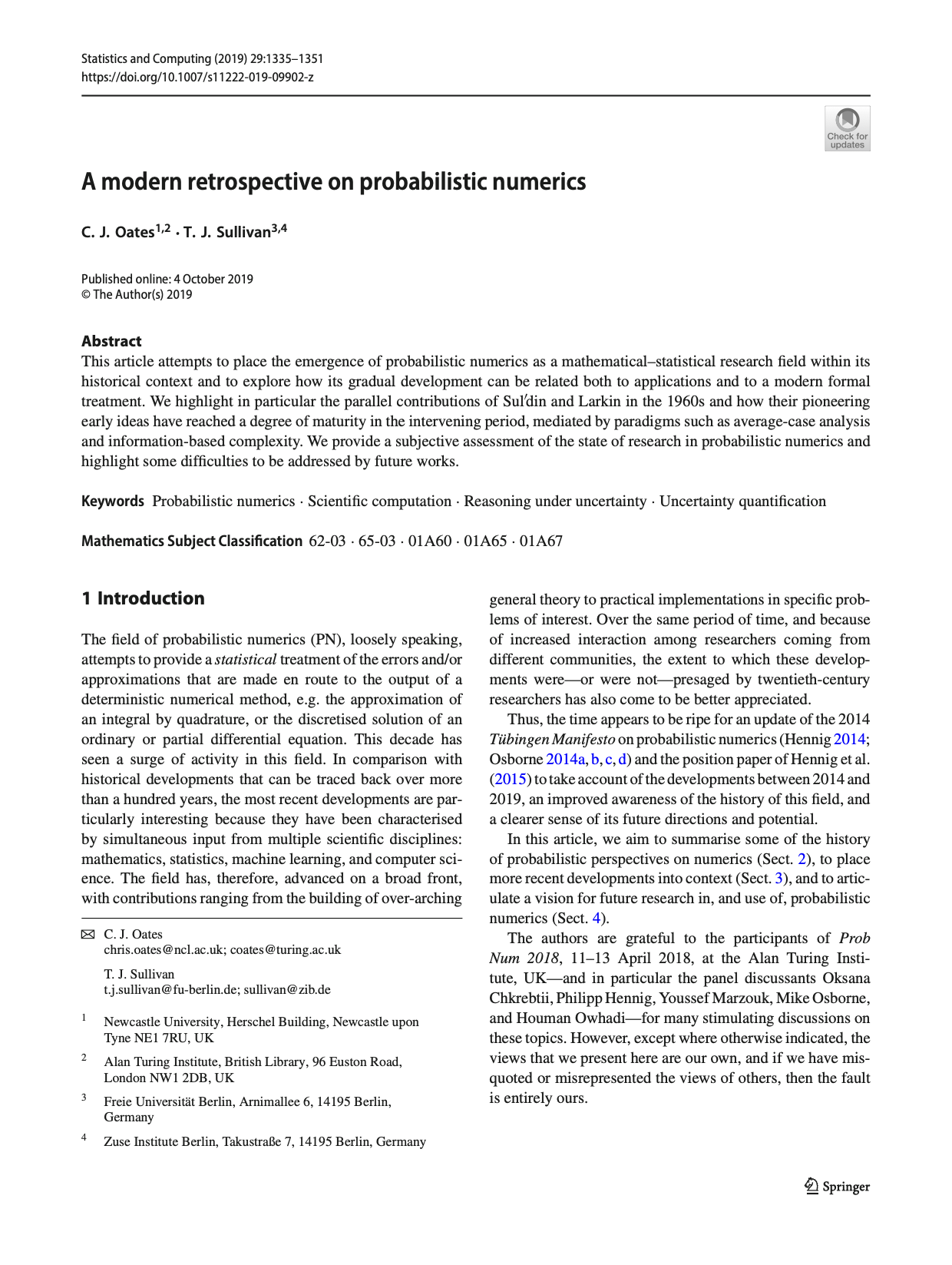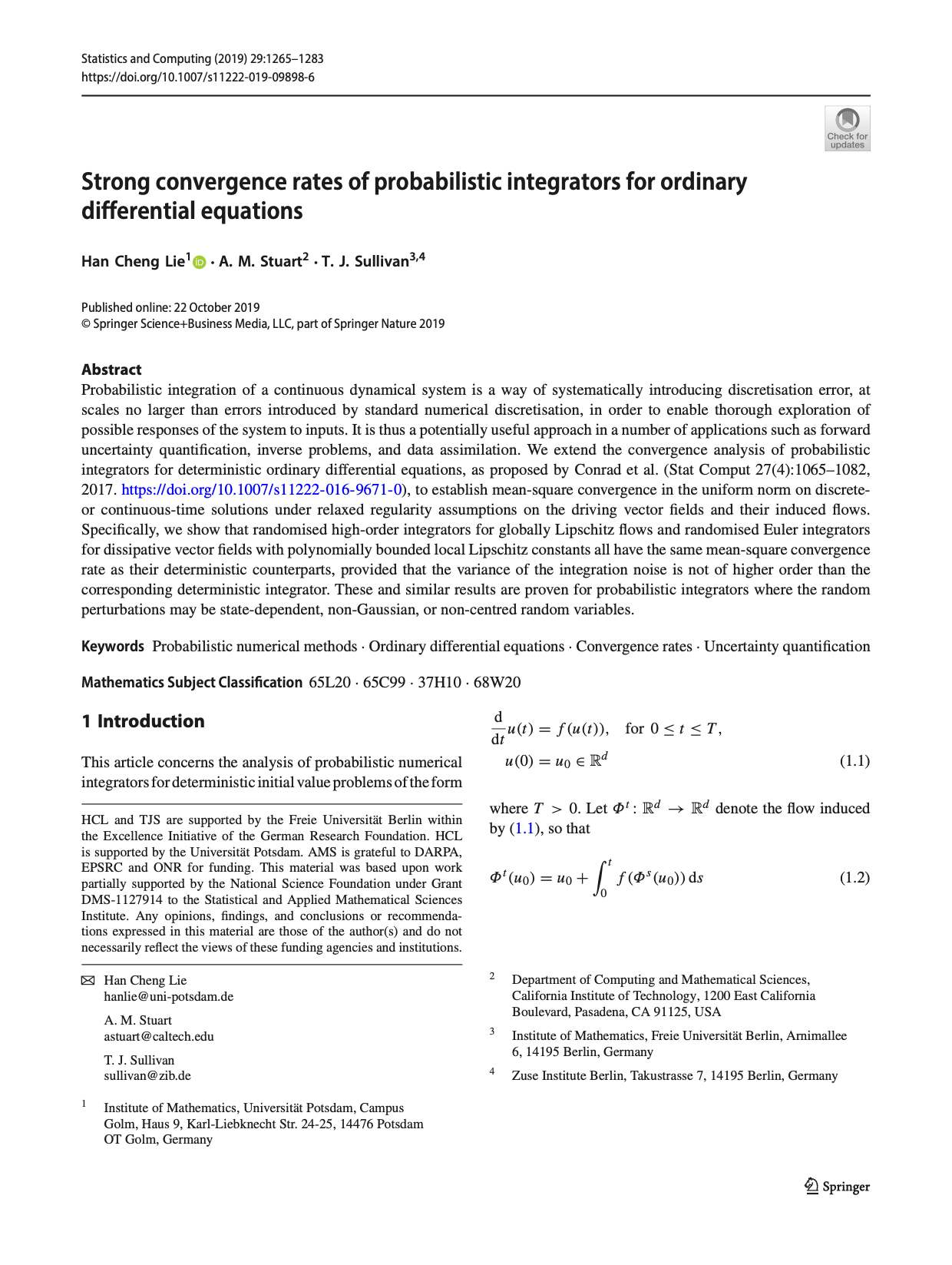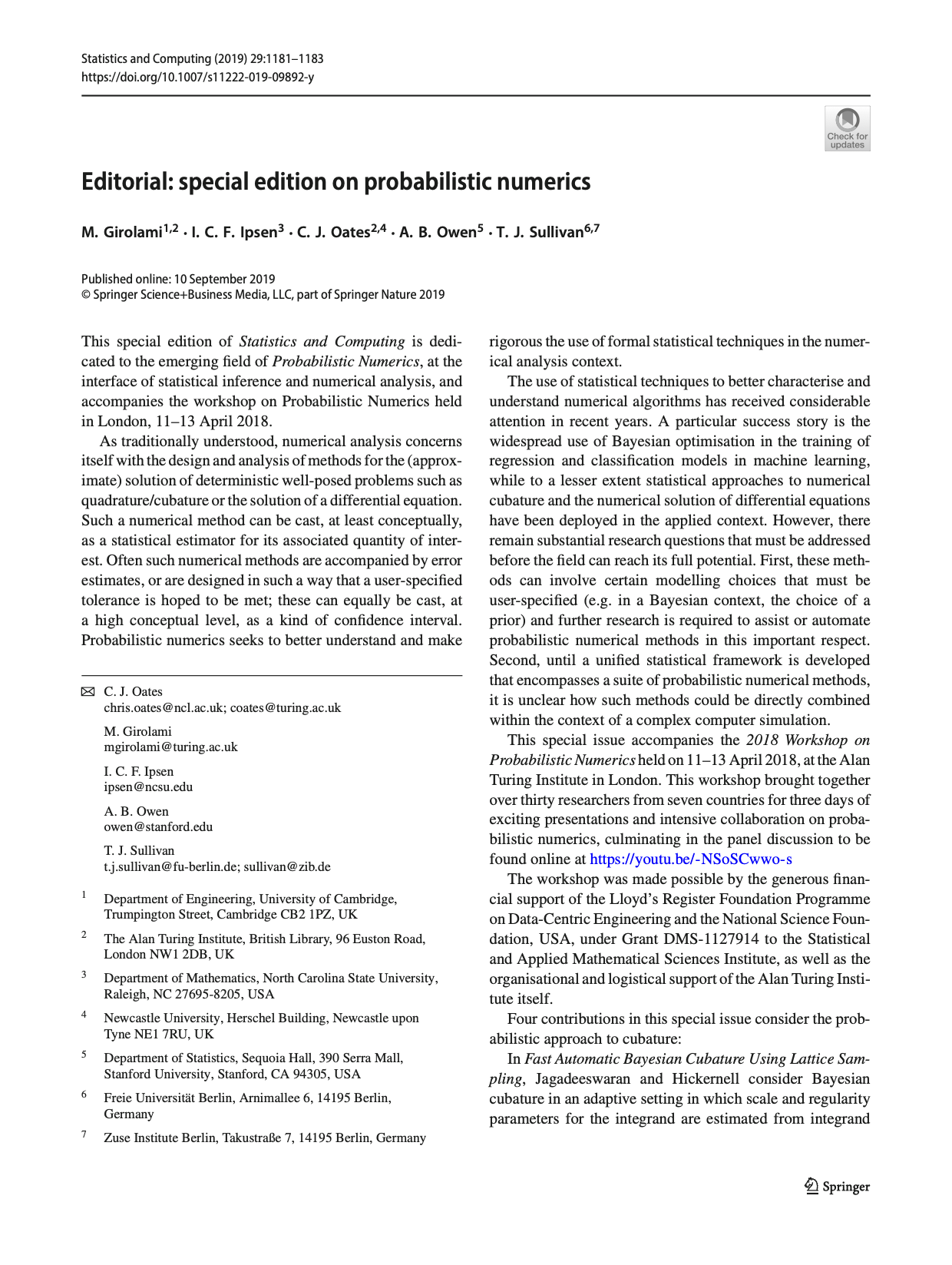# Tim Sullivan

### #stco

Clear Search### Convergence rates of Gaussian ODE filters in Statistics and Computing

The paper “Convergence rates of Gaussian ODE filters” by Hans Kersting, Philipp Hennig, and myself has just appeared in the journal Statistics and Computing. In this work, we examine the strong convergence rates of probabilistic solvers for ODEs of the form $$\dot{x}(t) = f(x(t))$$ that are based upon Gaussian filtering. In some sense, this work combines the numerical analysis perspective of Conrad et al. (2016) and Lie et al. (2019) with the filtering perspective on probabilistic numerical methods for ODEs of Schober et al. (2014).

H. Kersting, T. J. Sullivan, and P. Hennig. “Convergence rates of Gaussian ODE filters.” Statistics and Computing 30(6):1791–1816, 2020. doi:10.1007/s11222-020-09972-4

Abstract. A recently introduced class of probabilistic (uncertainty-aware) solvers for ordinary differential equations (ODEs) applies Gaussian (Kalman) filtering to initial value problems. These methods model the true solution $$x$$ and its first $$q$$ derivatives a priori as a Gauss–Markov process $$X$$, which is then iteratively conditioned on information about $$\dot{x}$$. This article establishes worst-case local convergence rates of order $$q + 1$$ for a wide range of versions of this Gaussian ODE filter, as well as global convergence rates of order $$q$$ in the case of $$q = 1$$ and an integrated Brownian motion prior, and analyses how inaccurate information on $$\dot{x}$$ coming from approximate evaluations of $$f$$ affects these rates. Moreover, we show that, in the globally convergent case, the posterior credible intervals are well calibrated in the sense that they globally contract at the same rate as the truncation error. We illustrate these theoretical results by numerical experiments which might indicate their generalizability to $$q \in \{ 2, 3 , \dots \}$$.

Published on Tuesday 15 September 2020 at 09:00 UTC #publication #stco #prob-num #kersting #hennig### Probabilistic numerics retrospective in Statistics and Computing

The special issue of Statistics and Computing dedicated to probabilistic numerics carries the article “A modern retrospective on probabilistic numerics” by Chris Oates and myself, which gives a historical overview of the field and some perspectives on future challenges.

C. J. Oates and T. J. Sullivan. “A modern retrospective on probabilistic numerics.” Statistics and Computing 29(6):1335–1351, 2019. doi:10.1007/s11222-019-09902-z

Abstract. This article attempts to place the emergence of probabilistic numerics as a mathematical–statistical research field within its historical context and to explore how its gradual development can be related both to applications and to a modern formal treatment. We highlight in particular the parallel contributions of Sul′din and Larkin in the 1960s and how their pioneering early ideas have reached a degree of maturity in the intervening period, mediated by paradigms such as average-case analysis and information-based complexity. We provide a subjective assessment of the state of research in probabilistic numerics and highlight some difficulties to be addressed by future works.

Published on Tuesday 5 November 2019 at 12:30 UTC #publication #stco #prob-num #oates### Strong convergence rates of probabilistic integrators for ODEs in Statistics and Computing

The special issue of Statistics and Computing dedicated to probabilistic numerics carries the article “Strong convergence rates of probabilistic integrators for ODEs” by Han Cheng Lie, Andrew Stuart, and myself on the convergence analysis of randomised perturbation-based time-stepping methods for the solution of ODE initial value problems.

H. C. Lie, A. M. Stuart, and T. J. Sullivan. “Strong convergence rates of probabilistic integrators for ordinary differential equations.” Statistics and Computing 29(6):1265–1283, 2019. doi:10.1007/s11222-019-09898-6

Abstract. Probabilistic integration of a continuous dynamical system is a way of systematically introducing discretisation error, at scales no larger than errors introduced by standard numerical discretisation, in order to enable thorough exploration of possible responses of the system to inputs. It is thus a potentially useful approach in a number of applications such as forward uncertainty quantification, inverse problems, and data assimilation. We extend the convergence analysis of probabilistic integrators for deterministic ordinary differential equations, as proposed by Conrad et al. (2016), to establish mean-square convergence in the uniform norm on discrete- or continuous-time solutions under relaxed regularity assumptions on the driving vector fields and their induced flows. Specifically, we show that randomised high-order integrators for globally Lipschitz flows and randomised Euler integrators for dissipative vector fields with polynomially bounded local Lipschitz constants all have the same mean-square convergence rate as their deterministic counterparts, provided that the variance of the integration noise is not of higher order than the corresponding deterministic integrator. These and similar results are proven for probabilistic integrators where the random perturbations may be state-dependent, non-Gaussian, or non-centred random variables.

Published on Tuesday 5 November 2019 at 12:00 UTC #publication #stco #prob-num #lie #stuart### Statistics and Computing special issue on Probabilistic Numerics

It is a great pleasure to announce that a special issue of Statistics and Computing (vol. 29, no. 6) dedicated to the theme of probabilistic numerics is now fully available online in print. This special issue, edited by Mark Girolami, Ilse Ipsen, Chris Oates, Art Owen, and myself, accompanies the 2018 Workshop on Probabilistic Numerics held at the Alan Turing Institute in London.

The special issue consists of a short editorial and ten full-length peer-reviewed research articles:

1. “De-noising by thresholding operator adapted wavelets” by G. R. Yoo and H. Owhadi
2. “Optimal Monte Carlo integration on closed manifolds” by M. Ehler, M. Gräf, and C. J. Oates
3. “Fast automatic Bayesian cubature using lattice sampling” by R. Jagadeeswaran and F. J. Hickernell
4. “Symmetry exploits for Bayesian cubature methods” by T. Karvonen, S. Särkkä, and C. J. Oates
5. “Probabilistic linear solvers: a unifying view” by S. Bartels, J. Cockayne, I. C. F. Ipsen, and P. Hennig
6. “Strong convergence rates of probabilistic integrators for ordinary differential equations” by H. C. Lie, A. M. Stuart, and T. J. Sullivan
7. “Adaptive step-size selection for state-space based probabilistic differential equation solvers” by O. A. Chkrebtii and D. A. Campbell
8. “Probabilistic solutions to ordinary differential equations as non-linear Bayesian filtering: A new perspective” by F. Tronarp, H. Kersting, S. Särkkä, and P. Hennig
9. “On the positivity and magnitudes of Bayesian quadrature weights” by T. Karvonen, M. Kanagawa, and S. Särkkä
10. “A modern retrospective on probabilistic numerics” by C. J. Oates and T. J. Sullivan

Published on Wednesday 30 October 2019 at 12:00 UTC #stco #prob-num #girolami #ipsen #oates #owen